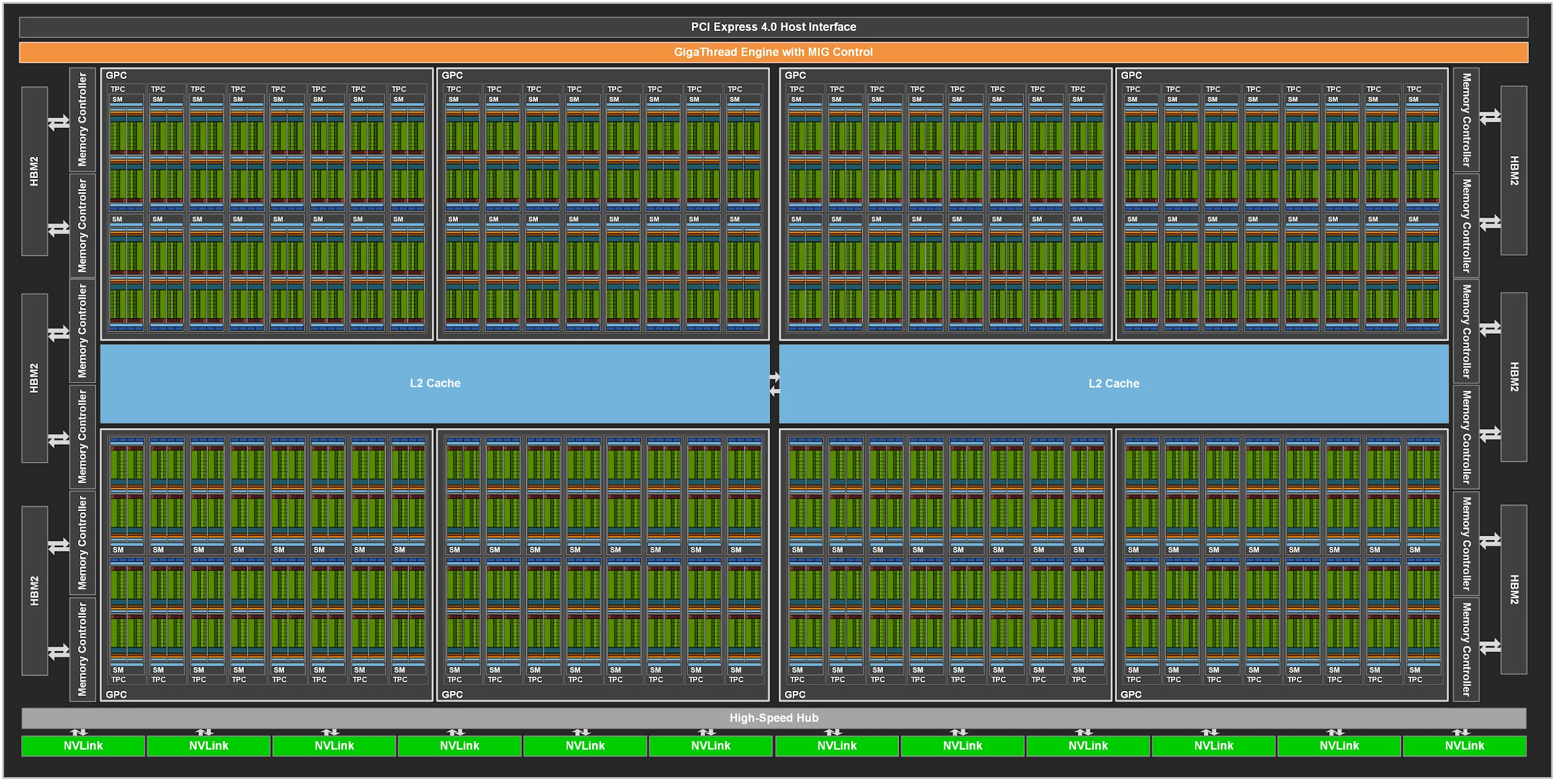# Math-Bound VS Memory-Bound Operations

## Introduction

In computer science, given a hardware, finding out the bottleneck of an operation is very critical for the performance. An operation could be either math-bound or memory-bound.

In this blog post, I would like to discuss the definitions of math-bound or memory-bound operations and some of the fundamental concepts in computer program optimization.

## Math Bandwidth

Math bandwidth the rate at which math unit operation can be conducted by a processor, and is usually expressed in units of operations/second (OPS). If the data being processed are floating point data, the unit FLOPS is more commonly used. The math bandwidth could be queried from the hardware specification, such as NVIDIA A100 GPU and Intel CPUs.

## Memory Bandwidth

Memory bandwidth is the rate at which data can be read from or stored into a semiconductor memory by a processor, and is usually expressed in units of bytes/second (B/s). The memory bandwidth could be queried from the hardware specification, such as NVIDIA A100 GPU, or computed theoretically, such as Intel Core-X CPUs.

## Data Reuse

Most processors, such as CPU and GPU, have cache. A cache is a smaller, faster memory, located closer to a processor core, which stores copies of the data from frequently used main memory locations. Accessing cache is much much faster than memory. Comparing with the time of accessing memory, the time of accessing cache is negligible. If some data need to be repeatedly used for a certain operation, it is always a good idea to copy the data to cache to avoid the slow memory access repeatedly. This is often called data reuse.Consider computing a matrix multiplication using two $N \times N$ matrices, resulting a new $N \times N$ matrix. It is not difficult to find that we have to do $N^3$ multiplications and $N^3$ additions, totaling $2N^3$ math operations, in order to compute the resulting matrix.

Suppose the value in the matrices is of $b$ bits, if there is no data reuse, we need to read the memory $2N \times N \times N = 2N^3$ times, and the amount of data read is $2bN^3$. With data reuse, if we can fit one of one $N \times N$ matrix and one $N$-sized array in cache to reuse, we only need to read $N \times N + N \times N = 2N^2$ values, and the amount of data read will $2bN^2$. This is also the minimum amount of the data read we have to perform. The amount of the data write is $bN^2$, which is the same for both with data reuse and without data reuse. Therefore, in the best scenario, the amount of data transfer we have to perform for matrix multiplication is $2bN^2 + bN^2 = 3bN^2$.

## Math-Bound VS Memory-Bound Operations

### Definitions of Math-Bound and Memory-Bound

Based on the number of math operations and memory accesses, math bandwidth, and memory bandwidth in the computer system, we could then calculate roughly the time required to perform the math operations and the memory accesses. Concretely,

$$\begin{gather} t_{\text{math}} = \frac{N_{\text{op}}}{\text{BW}_{\text{math}}} \\ t_{\text{mem}} = \frac{N_{\text{byte}}}{\text{BW}_{\text{mem}}} \\ \end{gather}$$

Consequently, the operation is math-bound if $t_{\text{math}} > t_{\text{mem}}$ and is memory-bound if $t_{\text{math}} < t_{\text{mem}}$.

Mathematically, it is also equivalent to say the operation is math-bound if

$$\begin{gather} \frac{N_{\text{op}}}{N_{\text{byte}}} > \frac{\text{BW}_{\text{math}}}{\text{BW}_{\text{mem}}} \end{gather}$$

and is memory-bound if

$$\begin{gather} \frac{N_{\text{op}}}{N_{\text{byte}}} < \frac{\text{BW}_{\text{math}}}{\text{BW}_{\text{mem}}} \end{gather}$$

### Improving Memory-Bound Operations to Math-Bound Operations

Usually we cannot change $N_{\text{op}}$ for an operation, meaning $N_{\text{op}}$ is usually a constant, but we can change $N_{\text{byte}}$ by reusing data. If an operation is memory-bound, this usually means the operation is under-performed or under-optimized and the computer system is under-utilized.

Looking back at the $N \times N$ matrix multiplication example we mentioned above, assuming the matrix multiplication implementation does not reuse data.

\begin{align} \frac{N_{\text{op}}}{N_{\text{byte}}} &= \frac{2N^3}{2bN^3 / 8} \\ &= \frac{8}{b} : \text{OP/byte} \\ \end{align}

If one of the matrices and an array are reused, we have

\begin{align} \frac{N_{\text{op}}}{N_{\text{byte}}} &= \frac{2N^3}{3bN^2 / 8} \\ &= \frac{16N}{3b} : \text{OP/byte} \\ \end{align}

This means reusing data reduces memory access and improves the operation performance. A memory-bound operation could be improved to math-bound operation by optimizing the reuse of data in the implementation.

Sometimes, enlarging the size of the operands may also improve the memory-bound operation to math-bound operation.

Looking back at the same $N \times N$ matrix multiplication example, assuming the datatype is $\text{FP32}$ ($b = 32$).

\begin{align} \frac{N_{\text{op}}}{N_{\text{byte}}} &= \frac{2N^3}{3bN^2 / 8} \\ &= \frac{16N}{3b} \\ &= \frac{N}{6} : \text{OP/byte} \\ \end{align}

If the operation is performed on NVIDIA A100 GPU, the math bandwidth is $19.5$ TFLOPS and the memory bandwidth for $\text{FP32}$ is $1.6$ TB/sec. Therefore,

$$\frac{\text{BW}_{\text{math}}}{\text{BW}_{\text{mem}}} = 12.2 : \text{OP/byte}$$

When $N >= 74$, the matrix multiplication operation is math-bound and when $N < 74$, the $N \times N$ matrix multiplication is memory-bound.

One small caveat here. Although for some operations, it is theoretically possible to turn the operation from memory-bound to math-bound by enlarging the size of the operands, because the reusable data size (cache size) on the hardware is limited, for some operations this strategy might not work in practice.